# Hi! determine the height of the mountain (using atmospheric pressure)

Krokodrile
Homework Statement:
determine the height of the mountain from the data shown. Consider the average air density of 0.074 lbm and g= 32 ft/s^2
Relevant Equations:
p = p*g*h
i have the first convertions of the atmospheric pressure:
1872 lbf/ft^2 = 89,632.6 Pa
2016 lbm/ft^2 = 96,325.4 Pa
g= 9.72 m/s^2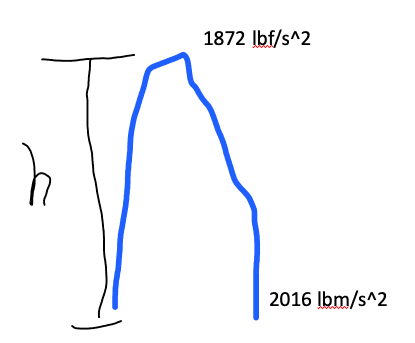But, i don't have idea how pass the air density of "lbm" to international units ;,(. And other cuestion: its fine pass lbm/ft^2 and lbf/ft^2 to Pa simirlarly?

Staff Emeritus
Homework Helper
Gold Member
The units are all over the place here. Is this the actual problem statement word for word and is the image from the problem?
• Density should have units of mass/length^3, but lbm is a unit of mass only.
• The units in the picture show lbf/s^2 and lbm/s^2 for the pressures. These are wrong as the dimensions of pressure are force/area while lbf/s^2 is force/time^2 and lbm/s^2 is mass/time^2.
I'd really recommend getting the input units correct before doing anything else.

•docnet
Krokodrile
The units are all over the place here. Is this the actual problem statement word for word and is the image from the problem?
• Density should have units of mass/length^3, but lbm is a unit of mass only.
• The units in the picture show lbf/s^2 and lbm/s^2 for the pressures. These are wrong as the dimensions of pressure are force/area while lbf/s^2 is force/time^2 and lbm/s^2 is mass/time^2.
I'd really recommend getting the input units correct before doing anything else.
yes, i wrong in the densty, its lbm/ft^3. yes, the units of pressures its lbf/ft^2 (top) and lbm/ft^2(bottom) (my mistake, sorry). But, i too have the question about these units: why lbf and lbm?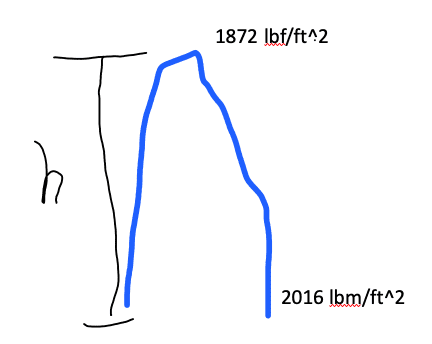Staff Emeritus
Homework Helper
Gold Member
Ok, the lbm/ft^2 is probably a mistake on the problem writer's part. It should likely be lbf/ft^2, which is the correct units for pressure (force/area).

Regarding your question on lbm, 1 lbm is defined as the mass for which the gravitational force in standard Earth gravity is 1 lbf. Since F = mg, 1 lbf = (1 lbm)g or in other words: 1 lbm = (1 lbf)/g. If you convert the 1 lbf to N and insert the appropriate value for g you will get the numerical value for 1 lbm expressed in kg. In order to get the SI units of kg/m^3 for the density, you will of course also have to convert the ft^3 to m^3.

•docnet
Krokodrile
Ok, the lbm/ft^2 is probably a mistake on the problem writer's part. It should likely be lbf/ft^2, which is the correct units for pressure (force/area).

Regarding your question on lbm, 1 lbm is defined as the mass for which the gravitational force in standard Earth gravity is 1 lbf. Since F = mg, 1 lbf = (1 lbm)g or in other words: 1 lbm = (1 lbf)/g. If you convert the 1 lbf to N and insert the appropriate value for g you will get the numerical value for 1 lbm expressed in kg. In order to get the SI units of kg/m^3 for the density, you will of course also have to convert the ft^3 to m^3.
I have all the units in international system, but i can't eliminate the s^2 of gravity and obtain m: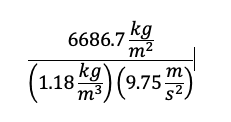Staff Emeritus
Homework Helper
Gold Member
The unit Pa is not kg/m^2 [mass/area], it is N/m^2 [force/area], which equates to (kg m/s^2)/m^2 = kg/(m s^2).

•docnet
Krokodrile
The unit Pa is not kg/m^2 [mass/area], it is N/m^2 [force/area], which equates to (kg m/s^2)/m^2 = kg/(m s^2).
Oohh, thanks for that, now i can eliminate the units, i don't know if i was bad because i got 1/m in the result: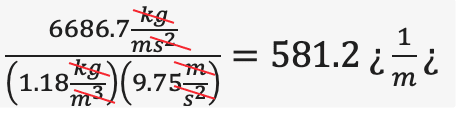Mentor
I would do this differently. 2016 - 1872 = 144 psf

144/0.084= 1714 ft

Krokodrile
I would do this differently. 2016 - 1872 = 144 psf

144/0.084= 1714 ft
in this case why the gravity was omited? and why 0.084 and not 0.074?

Mentor
in this case why the gravity was omited? and why 0.084 and not 0.074?
I misread the 0.074 as 0.084.
In imperial units, 1 lbm weighs 1 lbf. In these units, F = ma/g

Last edited:
Krokodrile
I misread the 0.074 as 0.084.
In imperial units, 1 lbm weighs 1 lbf.
I have difference of 11.92 m with my answer, i must check it again. The gravity was omited because the mountain its not afected by her? i don't understand why in 144/0.074 the gravity doesnt appears :(

Mentor
I have difference of 11.92 m with my answer, i must check it again. The gravity was omited because the mountain its not afected by her? i don't understand why in 144/0.074 the gravity doesnt appears :(
lbm is not the proper unit to use with such units . The correct unit to use is the slug, which is lbm/32.2. In this way, the 32.2’s cancel out.

Mentor
Suppose I defined 1 kgf as the weight of a 1 kg mass. How many Newton’s would that be?

Krokodrile
Suppose I defined 1 kgf as the weight of a 1 kg mass. How many Newton’s would that be?
9.80? as 1 kgf = 9.80N

Krokodrile
lbm is not the proper unit to use with such units . The correct unit to use is the slug, which is lbm/32.2. In this way, the 32.2’s cancel out.
if the slug 32.2s, its fine to cancel it with 32.0?

Mentor
if the slug 32.2s, its fine to cancel it with 32.0?
That was just rounded off to 32.

Krokodrile
That was just rounded off to 32.
then it would be something like this?: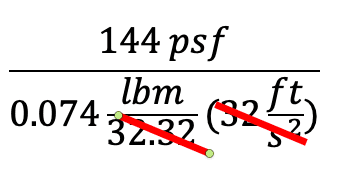Gordianus
Ah...The Imperial System...What was the name of that doomed satellite?

Krokodrile
Ah...The Imperial System...What was the name of that doomed satellite?
Mars Global Surveyor satellite?

Mentor
then it would be something like this?:
View attachment 280215
It should be $$\frac{144\ lb_f/ft^2}{0.074 \frac{lb_m}{ft^3}(32.2\ \frac{ft}{s^2})\left(\frac{1}{32.2\ \frac{lb_mft}{lb_fs^2}}\right)}$$

Krokodrile
It should be $$\frac{144\ lb_f/ft^2}{0.074 \frac{lb_m}{ft^3}(32.2\ \frac{ft}{s^2})\left(\frac{1}{32.2\ \frac{lb_mft}{lb_fs^2}}\right)}$$
thank you so much. I understand all the process, except for the 1/32: where does it come lbm/lb?

Mentor
thank you so much. I understand all the process, except for the 1/32: where does it come lbm/lb?
It is the factor that converts lbm to slugs. Those units are the same as lbm/slug

Krokodrile
It is the factor that converts lbm to slugs. Those units are the same as lb/slug
so, the ft/s^2 its the slug units and lbm/lb is the lfbm to slug factor?

Mentor
so, the ft/s^2 its the slug units and lbm/lb is the lfbm to slug factor?
$$32.2 lb_m=1\ slug= 1 \frac{lb_f s^2}{ft}$$

Krokodrile
$$32.2 lb_m=1\ slug= 1 \frac{lb_f s^2}{ft}$$
oooohhh, and 1/32 its because we can eliminate with the other 32. Ok, i get it. Thank you so much again for your time, Sir.

Staff Emeritus